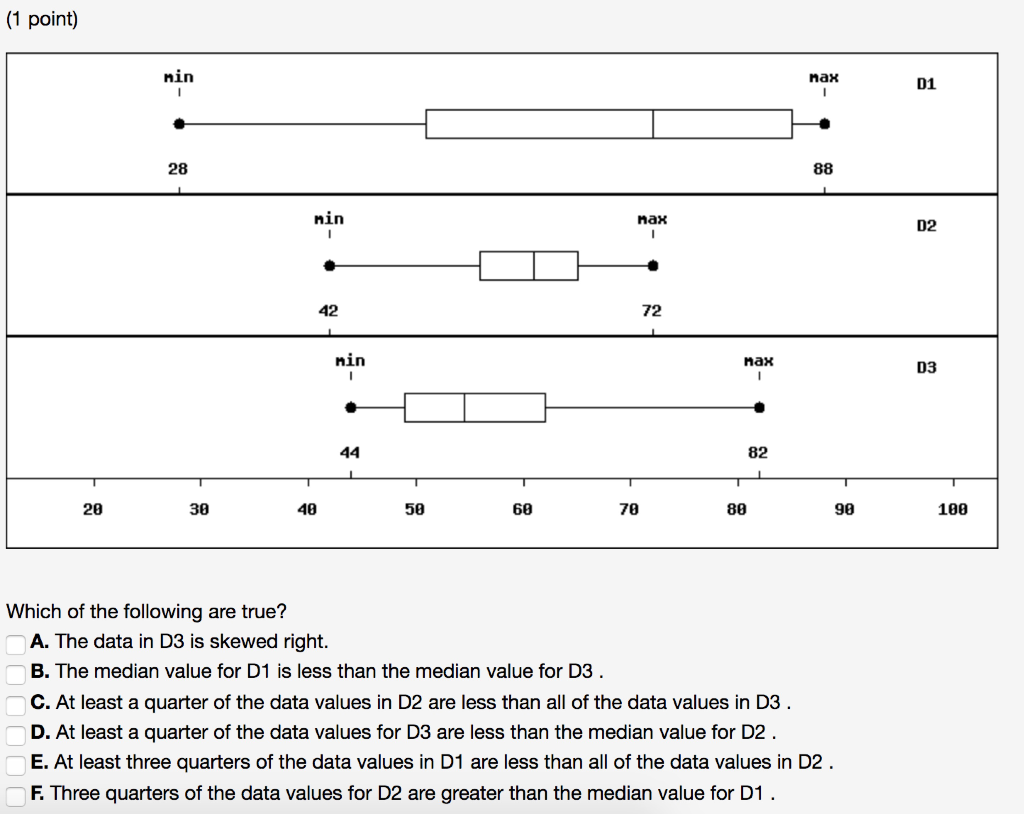Homework Help Question & Answers

(1 point) nin nax D1 28 Min ax D2 42 72 nin Max D3 82 20 30 48 50 60 70 80 90 180 Which of the fo...(1 point) nin nax D1 28 Min ax D2 42 72 nin Max D3 82 20 30 48 50 60 70 80 90 180 Which of the following are true? A. The data in D3 is skewed right B. The median value for D1 is less than the median value for D3 C. At least a quarter of the data values in D2 are less than all of the data values in D3 D. At least a quarter of the data values for D3 are less than the median value for D2 E. At least three quarters of the data values in D1 are less than all of the data values in D2 F. Three quarters of the data values for D2 are greater than the median value for D1

From the provided box plot:

Following statements are true.

A) The data in D3 is skewed right. Since it has long tail on right side.

C) At least quarter of the data values for D2 are less than all of the data the data values in D3.

Since Q1 and Q2 of D2 overlaps with Q3 and Q4 of D3.

D) At least quarter of the data values for D3 are less than the median value for D2.

Since the median for D3 is less than the Q1 of D2

Add Answer of: (1 point) nin nax D1 28 Min ax D2 42 72 nin Max D3 82 20 30 48 50 60 70 80 90 180 Which of the fo...
More Homework Help Questions Additional questions in this topic.

• (n-2)*180 =108n 180 n - 108 n = 360 72 n = 360 n = 5 drwls, I had totally misread the problem and thought it said (n-2)^180 =108n been working on it for almost an hour, trying Newton's method and all kinds of iteration

Need Online Homework Help?

Get FREE EXPERT Answers
WITHIN MINUTES
Related Questions